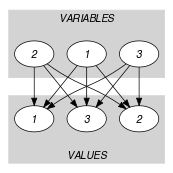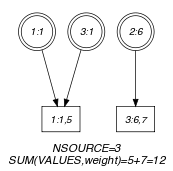## 5.388. sum_of_weights_of_distinct_values

Origin
Constraint

$\mathrm{𝚜𝚞𝚖}_\mathrm{𝚘𝚏}_\mathrm{𝚠𝚎𝚒𝚐𝚑𝚝𝚜}_\mathrm{𝚘𝚏}_\mathrm{𝚍𝚒𝚜𝚝𝚒𝚗𝚌𝚝}_\mathrm{𝚟𝚊𝚕𝚞𝚎𝚜}\left(\mathrm{𝚅𝙰𝚁𝙸𝙰𝙱𝙻𝙴𝚂},\mathrm{𝚅𝙰𝙻𝚄𝙴𝚂},\mathrm{𝙲𝙾𝚂𝚃}\right)$

Synonym

$\mathrm{𝚜𝚠𝚍𝚟}$.

Arguments
 $\mathrm{𝚅𝙰𝚁𝙸𝙰𝙱𝙻𝙴𝚂}$ $\mathrm{𝚌𝚘𝚕𝚕𝚎𝚌𝚝𝚒𝚘𝚗}\left(\mathrm{𝚟𝚊𝚛}-\mathrm{𝚍𝚟𝚊𝚛}\right)$ $\mathrm{𝚅𝙰𝙻𝚄𝙴𝚂}$ $\mathrm{𝚌𝚘𝚕𝚕𝚎𝚌𝚝𝚒𝚘𝚗}\left(\mathrm{𝚟𝚊𝚕}-\mathrm{𝚒𝚗𝚝},\mathrm{𝚠𝚎𝚒𝚐𝚑𝚝}-\mathrm{𝚒𝚗𝚝}\right)$ $\mathrm{𝙲𝙾𝚂𝚃}$ $\mathrm{𝚍𝚟𝚊𝚛}$
Restrictions
 $\mathrm{𝚛𝚎𝚚𝚞𝚒𝚛𝚎𝚍}$$\left(\mathrm{𝚅𝙰𝚁𝙸𝙰𝙱𝙻𝙴𝚂},\mathrm{𝚟𝚊𝚛}\right)$ $|\mathrm{𝚅𝙰𝙻𝚄𝙴𝚂}|>0$ $\mathrm{𝚛𝚎𝚚𝚞𝚒𝚛𝚎𝚍}$$\left(\mathrm{𝚅𝙰𝙻𝚄𝙴𝚂},\left[\mathrm{𝚟𝚊𝚕},\mathrm{𝚠𝚎𝚒𝚐𝚑𝚝}\right]\right)$ $\mathrm{𝚅𝙰𝙻𝚄𝙴𝚂}.\mathrm{𝚠𝚎𝚒𝚐𝚑𝚝}\ge 0$ $\mathrm{𝚍𝚒𝚜𝚝𝚒𝚗𝚌𝚝}$$\left(\mathrm{𝚅𝙰𝙻𝚄𝙴𝚂},\mathrm{𝚟𝚊𝚕}\right)$ $\mathrm{𝚒𝚗}_\mathrm{𝚊𝚝𝚝𝚛}$$\left(\mathrm{𝚅𝙰𝚁𝙸𝙰𝙱𝙻𝙴𝚂},\mathrm{𝚟𝚊𝚛},\mathrm{𝚅𝙰𝙻𝚄𝙴𝚂},\mathrm{𝚟𝚊𝚕}\right)$ $\mathrm{𝙲𝙾𝚂𝚃}\ge 0$
Purpose

All variables of the $\mathrm{𝚅𝙰𝚁𝙸𝙰𝙱𝙻𝙴𝚂}$ collection take a value in the $\mathrm{𝚅𝙰𝙻𝚄𝙴𝚂}$ collection. In addition $\mathrm{𝙲𝙾𝚂𝚃}$ is the sum of the $\mathrm{𝚠𝚎𝚒𝚐𝚑𝚝}$ attributes associated with the distinct values taken by the variables of $\mathrm{𝚅𝙰𝚁𝙸𝙰𝙱𝙻𝙴𝚂}$.

Example
$\left(\begin{array}{c}〈1,6,1〉,\hfill \\ 〈\begin{array}{cc}\mathrm{𝚟𝚊𝚕}-1\hfill & \mathrm{𝚠𝚎𝚒𝚐𝚑𝚝}-5,\hfill \\ \mathrm{𝚟𝚊𝚕}-2\hfill & \mathrm{𝚠𝚎𝚒𝚐𝚑𝚝}-3,\hfill \\ \mathrm{𝚟𝚊𝚕}-6\hfill & \mathrm{𝚠𝚎𝚒𝚐𝚑𝚝}-7\hfill \end{array}〉,12\hfill \end{array}\right)$

The $\mathrm{𝚜𝚞𝚖}_\mathrm{𝚘𝚏}_\mathrm{𝚠𝚎𝚒𝚐𝚑𝚝𝚜}_\mathrm{𝚘𝚏}_\mathrm{𝚍𝚒𝚜𝚝𝚒𝚗𝚌𝚝}_\mathrm{𝚟𝚊𝚕𝚞𝚎𝚜}$ constraint holds since its last argument $\mathrm{𝙲𝙾𝚂𝚃}=12$ is equal to the sum $5+7$ of the weights of the values 1 and 6 that occur within the $〈1,6,1〉$ collection.

Typical
 $|\mathrm{𝚅𝙰𝚁𝙸𝙰𝙱𝙻𝙴𝚂}|>1$ $\mathrm{𝚛𝚊𝚗𝚐𝚎}$$\left(\mathrm{𝚅𝙰𝚁𝙸𝙰𝙱𝙻𝙴𝚂}.\mathrm{𝚟𝚊𝚛}\right)>1$ $|\mathrm{𝚅𝙰𝙻𝚄𝙴𝚂}|>1$ $\mathrm{𝚛𝚊𝚗𝚐𝚎}$$\left(\mathrm{𝚅𝙰𝙻𝚄𝙴𝚂}.\mathrm{𝚠𝚎𝚒𝚐𝚑𝚝}\right)>1$ $\mathrm{𝚖𝚊𝚡𝚟𝚊𝚕}$$\left(\mathrm{𝚅𝙰𝙻𝚄𝙴𝚂}.\mathrm{𝚠𝚎𝚒𝚐𝚑𝚝}\right)>0$
Symmetries
• Items of $\mathrm{𝚅𝙰𝚁𝙸𝙰𝙱𝙻𝙴𝚂}$ are permutable.

• All occurrences of two distinct values of $\mathrm{𝚅𝙰𝚁𝙸𝙰𝙱𝙻𝙴𝚂}.\mathrm{𝚟𝚊𝚛}$ can be swapped.

• Items of $\mathrm{𝚅𝙰𝙻𝚄𝙴𝚂}$ are permutable.

• All occurrences of two distinct values in $\mathrm{𝚅𝙰𝚁𝙸𝙰𝙱𝙻𝙴𝚂}.\mathrm{𝚟𝚊𝚛}$ or $\mathrm{𝚅𝙰𝙻𝚄𝙴𝚂}.\mathrm{𝚟𝚊𝚕}$ can be swapped; all occurrences of a value in $\mathrm{𝚅𝙰𝚁𝙸𝙰𝙱𝙻𝙴𝚂}.\mathrm{𝚟𝚊𝚛}$ or $\mathrm{𝚅𝙰𝙻𝚄𝙴𝚂}.\mathrm{𝚟𝚊𝚕}$ can be renamed to any unused value.

Arg. properties

Functional dependency: $\mathrm{𝙲𝙾𝚂𝚃}$ determined by $\mathrm{𝚅𝙰𝚁𝙸𝙰𝙱𝙻𝙴𝚂}$ and $\mathrm{𝚅𝙰𝙻𝚄𝙴𝚂}$.

attached to cost variant: $\mathrm{𝚗𝚟𝚊𝚕𝚞𝚎}$ (all values have a weight of 1).

Keywords
Arc input(s)

$\mathrm{𝚅𝙰𝚁𝙸𝙰𝙱𝙻𝙴𝚂}$ $\mathrm{𝚅𝙰𝙻𝚄𝙴𝚂}$

Arc generator
$\mathrm{𝑃𝑅𝑂𝐷𝑈𝐶𝑇}$$↦\mathrm{𝚌𝚘𝚕𝚕𝚎𝚌𝚝𝚒𝚘𝚗}\left(\mathrm{𝚟𝚊𝚛𝚒𝚊𝚋𝚕𝚎𝚜},\mathrm{𝚟𝚊𝚕𝚞𝚎𝚜}\right)$

Arc arity
Arc constraint(s)
$\mathrm{𝚟𝚊𝚛𝚒𝚊𝚋𝚕𝚎𝚜}.\mathrm{𝚟𝚊𝚛}=\mathrm{𝚟𝚊𝚕𝚞𝚎𝚜}.\mathrm{𝚟𝚊𝚕}$
Graph property(ies)
 $•$$\mathrm{𝐍𝐒𝐎𝐔𝐑𝐂𝐄}$$=|\mathrm{𝚅𝙰𝚁𝙸𝙰𝙱𝙻𝙴𝚂}|$ $•$$\mathrm{𝐒𝐔𝐌}$$\left(\mathrm{𝚅𝙰𝙻𝚄𝙴𝚂},\mathrm{𝚠𝚎𝚒𝚐𝚑𝚝}\right)=\mathrm{𝙲𝙾𝚂𝚃}$

Signature

Since we use the $\mathrm{𝑃𝑅𝑂𝐷𝑈𝐶𝑇}$ arc generator, the number of sources of the final graph cannot exceed the number of sources of the initial graph. Since the initial graph contains $|\mathrm{𝚅𝙰𝚁𝙸𝙰𝙱𝙻𝙴𝚂}|$ sources, this number is an upper bound of the number of sources of the final graph. Therefore we can rewrite $\mathrm{𝐍𝐒𝐎𝐔𝐑𝐂𝐄}=|\mathrm{𝚅𝙰𝚁𝙸𝙰𝙱𝙻𝙴𝚂}|$ to $\mathrm{𝐍𝐒𝐎𝐔𝐑𝐂𝐄}\ge |\mathrm{𝚅𝙰𝚁𝙸𝙰𝙱𝙻𝙴𝚂}|$ and simplify $\underline{\overline{\mathrm{𝐍𝐒𝐎𝐔𝐑𝐂𝐄}}}$ to $\overline{\mathrm{𝐍𝐒𝐎𝐔𝐑𝐂𝐄}}$.

Parts (A) and (B) of Figure 5.388.1 respectively show the initial and final graph associated with the Example slot. Since we use the $\mathrm{𝐍𝐒𝐎𝐔𝐑𝐂𝐄}$ graph property, the source vertices of the final graph are shown in a double circle. Since we also use the $\mathrm{𝐒𝐔𝐌}$ graph property we show the vertices from which we compute the total cost in a box.

##### Figure 5.388.1. Initial and final graph of the $\mathrm{𝚜𝚞𝚖}_\mathrm{𝚘𝚏}_\mathrm{𝚠𝚎𝚒𝚐𝚑𝚝𝚜}_\mathrm{𝚘𝚏}_\mathrm{𝚍𝚒𝚜𝚝𝚒𝚗𝚌𝚝}_\mathrm{𝚟𝚊𝚕𝚞𝚎𝚜}$ constraint(a) (b)